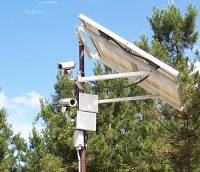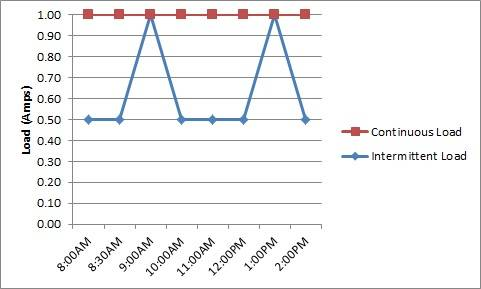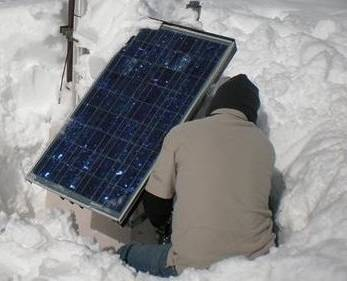## How to Calculate Loads for Off-Grid Systems

In solar terminology, the term “load” refers to the power consumption of the device(s) that are being used in the system. Understanding your loads is critical to maintaining a well functioning power system, as we will explain in this article. Many of the customer issues SunWize sees are not because of anything to do with the power system itself, but because customer’s don’t understand their own loads!!For example, an off-grid solar system may power a security camera, a radio, or both! The power being consumed by these devices are the system’s “loads”. The security camera may consume 10W of power, while the radio may consume 12W of power, resulting in a total continuous load of 22W, if using both simultaneously, which of course is very straightforward!

## Reasons a 100W module cannot run a 100W load:

6. The module rating is lab created
5. Storms happen
4. Season effective disorder (there is less sun light in the winter)
3. Night comes every day
2. If it did you wouldn’t need batteries for storage
1. Because it just doesn’t work

Properly calculating load consumption is a critical step in properly sizing an off-grid power system. As most off-grid systems are dependent on batteries to store energy, and these battery capacities are sized based on amp hours, the load needs to be calculated in amp hours in order to properly size the system. You can learn more about this in our basic system sizing webinar!

In this Tech-Note we’ll cover several different types of loads and how to properly convert them into amp hours. All loads can be defined as the following:• continuous or non-continuous
• at system voltage or at non-system voltage.

Continuous is defined as 24 hours a day, 7 days a week. Non-continuous, also referred to as intermittent, defines a load that operates for a portion of a day, or has different consumption at different times of the day. Typical non-continuous loads are lighting, radios on transmit, valves or actuators, pumps or motors, gate openers, etc.

## Temperature Compensation

Because off-grid systems typically use lead-acid batteries as a storage mechanism, the system voltage can vary from -10% to 20% of its nominal value. As an example, a nominal 24VDC system can vary from 21.6VDC to 28.8VDC or even higher in colder climates with temperature compensation. It is important to understand this operating voltage is for the health of the battery and NOT for the load.• Although the minimum system voltage can be adjusted higher this will prevent the system from being able to utilize the full capacity of the battery.
• Adjusting the minimum system voltage lower will cause the battery to be over-discharged and possibly damaged.
• The maximum system voltage is set to properly recharge the battery, adjusting this voltage higher will over-charge the battery causing a VRLA to gas and reduce its capacity.
• Adjusting the maximum system voltage lower will cause the battery to not be fully charged. If a lead-acid battery is not periodically fully charged, sulfation crystals will form on the plates causing the battery to lose capacity and fail prematurely.

Note: the maximum system voltage is further adjusted for temperature, higher in temperatures below 25°C and lower in temperatures above 25°C. This is referred to as temperature compensation and is important for the battery to be properly charged. For more information on temperature compensation refer to the tech note by clicking here.

Now that we understand a little bit about the range and limitations of what “nominal battery voltage” means, how does that relate to our loads?

If the load cannot operate within the voltage tolerance of the system, then power conditioning equipment will be required. Typical power conditioning equipment would be a DC to AC inverter for AC loads, or a DC to DC converter for DC loads. Power conditioning equipment contains losses that need to be included in the load calculation. Typical efficiencies are 85-95% for inverters and 80-90% for converters.

Loads can be expressed in any of the formats listed below:

• current (amps[A])
• power (watts[W], kilowatts[kW])
• energy (amp hours[Ah], watthours[Wh], or kilowatthours[kWh]).

Notice, most utility bills are based on kWh, so this is a common unit for AC loads. Regardless of how the load is expressed, it will need to be converted to Ah for system sizing calculations. Recall from the power equations [Power(W) = Current(A)*Voltage(V)] we can convert from Watts to Amps by dividing power by nominal voltage [Power(W)/Voltage(V) = Current(A)].

Some load calculations will be simpler than others depending on the type of load and application. Ultimately, all answers will be expressed in the total amount of Amp-Hours consumed over a full day (24-hour period), for a given nominal voltage (Ah at 12V vs Ah at 24V are different things).

Let’s begin with the simplest example:
The simplest load is one that is:

• expressed in amps and
• is continuous and
• will operate within system voltage.

To calculate the load in this example you simply multiply the amps by 24 hours (the number of hours in a day) to get the daily load in Amp-Hours, often abbreviated “Ah”.Example 1:
0.5A x 24h/day = 12Ah/day @ 12VDC

If that same load were expressed in watts instead of amps, we would just convert it to amps by dividing power(W) by nominal voltage (V) and follow the same procedure.

Example 2:
6W/12VDC = 0.5A x 24h/day = 12Ah/day @ 12VDC

If the load is non-continuous, we simply multiply it by the number of hours per day it is active rather than by 24.Example 3:
8W, 12VDC LED light that operates only at night.

Let’s assume worse case the light will operate for 16 hours in the winter.

8W/12VDC = 0.67A x 16h/day = 10.67Ah/day @12VDC

Example 4:
A 12VDC radio that is 1A in standby and 10A in transmit. The radio transmits for 1 hour per day.
10A x 1h/day + 1A x 23h/day = 10Ah/day + 23Ah/day = 33Ah/day @12VDCExample 5:
A 10A, 24VDC valve that operates for 15 minutes a day and a 20W, 24VDC continuous controller.
10A x 0.25h/day + 20W/24VDC x 24h/day = 2.5Ah/day + 20Ah/day = 22.5Ah/day @ 24VDC

If the load is at voltage other than the system voltage then the losses of the conversion equipment need to be included in the calculations.

Example 6:
8W, 120VAC LED light that operates only at night.

Let’s assume worse case the light will operate for 16 hours in the winter.

Let’s also assume a system voltage of 12VDC and a power conversion efficiency of 90%.

8W/90%/12VDC = 0.74A x 16h/day = 11.85Ah/day @ 12VDCExample 7:
5W, 24VDC continuous sensors with a voltage tolerance of +/-10%.

As the load has a small voltage tolerance, a DC/DC converter will be required, as 24V +-2.4V is much narrower than the 21.6V to 28.8V we determined at the beginning may be present from a nominal 24V battery voltage.

Let’s assume the converter efficiency is 80% and the system voltage will be 24VDC.

5W/80%/24VDC = 0.26A x 24h/day = 6.25Ah/day @ 24VDC

If there are multiple loads, they can be calculated separately and then summed together to get the total load.Example 8:
Telecommunications tower with a 500W, 48VDC continuous communication load and 50W 24VDC nighttime obstruction lighting load.

Again, let’s assume the worst case for the lighting load is 16 hours in the winter. We’ll also assume a DC:DC Converter will be required for the 24VDC load, with an efficiency of 85%.